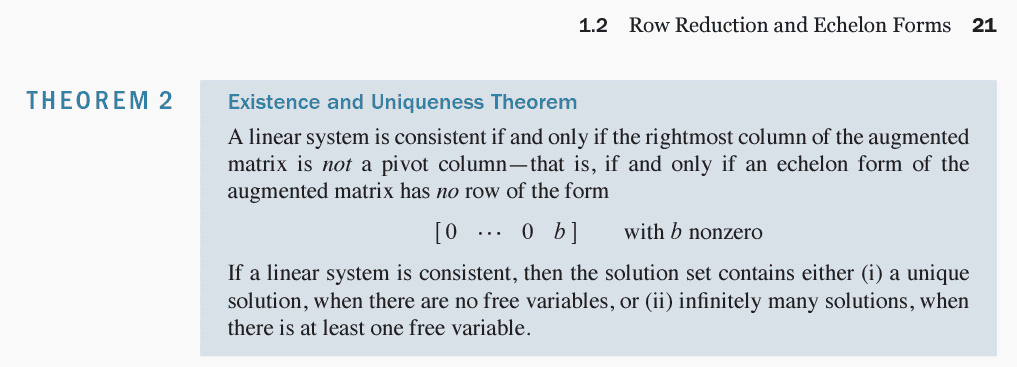# 311.1.7.9 For what values of h is v_3 in Span {v_1,v_2,v_3}

• MHB
• karush

#### karush

Gold Member
MHB
$\tiny{311.1.7.9}$
For what values of $h$ is $v_3$ in Span $\{v_1,v_2,v_3\}$ linearly \textit{dependent}. Justify
$v_1=\left[\begin{array}{rrrrrr}1\\-3\\2\end{array}\right], v_2=\left[\begin{array}{rrrrrr}-3\\9\\-6\end{array}\right], v_3=\left[\begin{array}{rrrrrr}5\\-7\\h\end{array}\right]$

$v_3$ is in Span{v1, v2} means there exists a constant such that

$c_1v_1 + c_2v_2 = v_3$

So if, but this is an augmented matrix

$\left[\begin{array}{rr|r}1 &−3& 2 \\ −3 &9 &−7 \\5 &−7& h \end{array}\right]$
RREF
$\left[ \begin{array}{ccc} 1 & -3 & 2 \\0 & 0 & -1 \\0 & 8 & h - 10 \end{array} \right]$
anyway, so far:unsure:$Last edited: ## Answers and Replies Your wording is a little off- it is not a vector in the span that is independent, it is the set of vectors themselves. And to do this problem you need to think about the DEFNITION of "independent vectors": these vectors are independent if and only there do NOT exist numbers, a, b, and c, not all 0, such that $$\displaystyle a\begin{bmatrix}1 \\ -2 \\ 3\end{bmatrix}+ b\begin{bmatrix}-3 \\ 9 \\ -6 \end{bmatrix}+ c \begin{bmatrix}5 \\ -7 \\ h \end{bmatrix}= \begin{bmatrix} 0 \\ 0 \\ 0 \end{bmatrix}$$ That is the same as saying a- 3b+ 5c= 0 -2a+ 9b- 7c= 0 3a- 6b+ hc= 0 Try to solve those. h will make those vectors independent if the only solution is a= b= c= 0, That can be done using an augmented matrix similar to your matrix- but your matrix is not "augmented". You need\begin{bmatrix}1 & -3 & 5 & 0 \\ -2 & 9 & -7 & 0 \\ 3 & -6 & h & 0 \end{bmatrix}. ok here is the theorm I think we need r3 is a pivit rowLast edited: Starting from$\begin{bmatrix}1 & -3 & 5 & 0 \\ -2 & 9 & -7 & 0 \\ 3 & -6 & h & 0 \end{bmatrix}$add twice the first row to the second row and subtract three times the first row from the third row to get$\begin{bmatrix}1 & -3 & 5 & 0 \\ 0 & 3 & 3 & 0 \\ 0 & 3 & h-15 & 0 \end{bmatrix}$. Now subtract the new second row from the third row to get$\begin{bmatrix}1 & -3 & 5 & 0 \\ 0 & 3 & 3 & 0 \\ 0 & 0 & h-18 & 0 \end{bmatrix}\$.

That matrix is equivalent to the equations a- 3b+ 5c= 0, 3b+ 3c= 0, and (h- 18)c= 0. If h is not 18, we can divide both side of the last equation by h- 18 to get c= 0. Then the second equation becomes 3b= 0 so b= 0 and the first equation becomes a= 0. That is, if h is anything other than 18, the vectors are independent. If h= 18, however, (h- 18)c= 0 becomes 0= 0 which is true for any c, 3b+ 3c= 0 gives b= -c and a- 3b+ 5c= a+ 8c= 0 so a= -8c. There exist infinitely many a, b, c that make the equations true so the vectors are dependent.

I don't know why you are giving the definition of a system of equations being "consistent". The exercise in your first post says nothing about a system of equation nor "consistency".